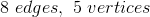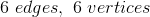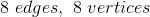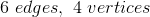# GED Math : Edges and Vertices

## Example Questions

### Example Question #1641 : Ged Math

How many edges and vertices are found on a square pyramid?Explanation:

The base of a square pyramid is, as the name suggests, a square which hasedges andvertices. The vertices of the square each have edges that meet at a single point, adding an additional vertex andadditional edges. Together, a square pyramid hasedges andvertices.

### Example Question #121 : 3 Dimensional Geometry

How many vertices does an octagonal pyramid have?

Six

Eight

Nine

Seven